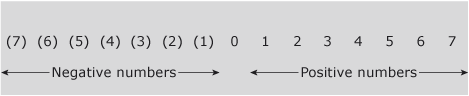### Become an OU studentIntroduction to bookkeeping and accounting

Start this free course now. Just create an account and sign in. Enrol and complete the course for a free statement of participation or digital badge if available.

# 1.7 Negative numbers and the use of brackets

Numbers smaller than zero (shown to the left of zero on the number line in the figure below) are called negative numbers. We indicate they are negative by putting them in brackets as shown in the figure below.Figure 4

Rules of negative numbers

The rules for using negative numbers can be summarised as follows:

• Adding a negative number is the same as subtracting a positive 50 + (-30) = 50 – 30 = 20
• Subtracting a negative number is the same as adding a positive 50 – (-30) = 50 + 30 = 80

Multiplication and division

• A positive number multiplied by a negative gives a negative 20 x -4 = -80
• A positive number divided by a negative gives a negative 20 / -4 = -5
• A negative number multiplied by a negative gives a positive -20 x -4 = 80
• A negative number divided by a negative gives a positive -20 / -4 = 5

Try to confirm the above rules for yourself by carrying out the following exercise either manually or by means of a calculator.

## Activity 9

Calculate each of the following. (In this activity we will assume the convention that if a number is in brackets it means it is negative).

(a) (2) x (3)

(a) 6

(b) 6 – (8)

(b) 14

(c) 6 + (8)

(c) (2)

(d) 2 x (3)

(d) (6)

(e) (8) / 4

(e) (2)

(f) (8) / (4)